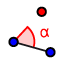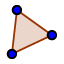# Angle-Side-Angle

## ASA Explained

If two angles and the included side of one triangle are congruent to the corresponding parts of another triangle, the triangles are congruent. The "included side" in ASA is the side between the angles being used. It is the side where the rays of the angles overlap. Below is an example how to construct this. If you change anything in the construction, just click on the arrows on the top right to restore the construction.

## Steps in constructing ASA

Now you try to draw a triangle congruent to the previous one﻿ You need to draw a triangle with side AB=8cm included between an angle CAB of 40 degrees and angle CBA of 30 degrees. Try to do this in the "Applet" below

1. Useto draw segment AB and if you are requested to give the length type in 5
2. Useto draw an angle at point A. (Hint: Always click last on the point where you want the angle.) If requested for the angle size type in 40 degrees. Lastly you need to select clockwise or counterclockwise. The direction of movement is from the line in a clockwise or counterclockwise direction.
3. Useto draw a ray from point A through point B' that were created by the angle tool.
4. Useto draw an angle at point B. If requested for the angle size type in 30 degrees.
5. Useto draw a ray from point B through point A' that were created by the angle tool.
6. Useto place point C at the intersection of the two rays
7. Useto draw triangle ABC